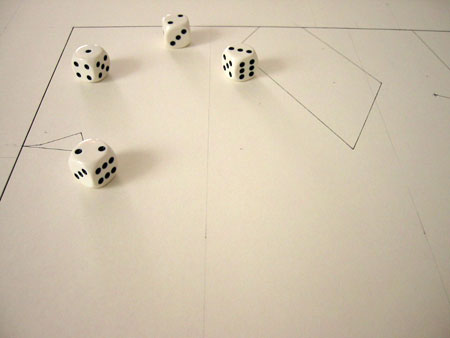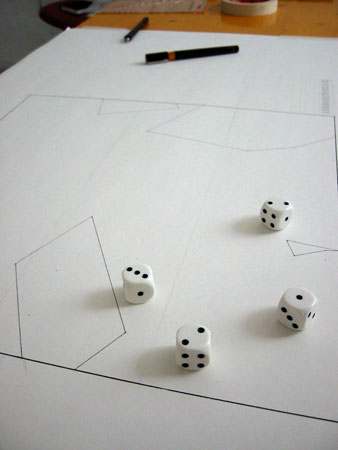# dé à dessiner

Manual drawing program

This project was presented in the Hotel Pupik (Austria) during Summer '05.Requirement: 1 sheet of paper, 4 dice

Algorithm:
```	Start:
draw the first point by throwing dice {d1, d2, d3, d4}
x = d1 * d2
y = d3 * d4

Loop: throw dice until a previous combination comes up
add the next point and draw the line between  the two last points
x = d1 * f(d2)
y = d3 * f(d4)

where:
f(1) = - 2	f(4) = + 0.5
f(2) = - 1	f(5) = + 1
f(3) = - 0.5	f(6) = + 2
```
The drawings: (click to enlarge)

Notes: à dessiner is a program using the human machine to generate drawings.
The time required to design may vary between 2 hours and 7 hours. The resulting drawing may simple or pretty complex. 6^4 = 1296 dice combinaisons are available. The probability to get a double is around 51 steps. Dé à dessiner is also a tribute to Mallarmé poem "Un coup de dés jamais n'abolira le hasard"